# Simulate from Existing Data

#### 2023-04-18

library(ggplot2)
library(dplyr)
library(tidyr)
library(faux)

The sim_df() function produces a data table with the same distributions and correlations as an existing data table. It simulates all numeric variables from a continuous normal distribution (for now).

For example, here is the relationship between speed and distance in the built-in dataset cars.

cars %>%
ggplot(aes(speed, dist)) +
geom_point() +
geom_smooth(method = "lm", formula = "y~x")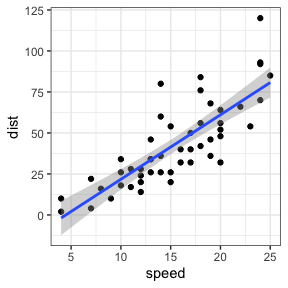Original cars dataset

You can create a new sample with the same parameters and 500 rows with the code sim_df(cars, 500).

sim_df(cars, 500) %>%
ggplot(aes(speed, dist)) +
geom_point() +
geom_smooth(method = "lm", formula = "y~x")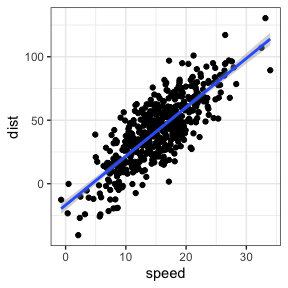Simulated cars dataset

## Between-subject variables

You can also optionally add between-subject variables. For example, here is the relationship between horsepower (hp) and weight (wt) for automatic (am = 0) versus manual (am = 1) transmission in the built-in dataset mtcars.

mtcars %>%
mutate(transmission = factor(am, labels = c("automatic", "manual"))) %>%
ggplot(aes(hp, wt, color = transmission)) +
geom_point() +
geom_smooth(method = "lm", formula = "y~x")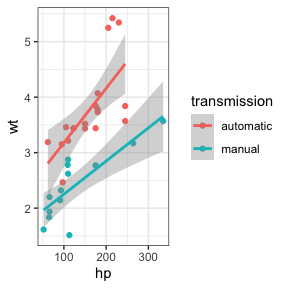Original mtcars dataset

And here is a new sample with 50 observations of each.

sim_df(mtcars, 50 , between = "am") %>%
mutate(transmission = factor(am, labels = c("automatic", "manual"))) %>%
ggplot(aes(hp, wt, color = transmission)) +
geom_point() +
geom_smooth(method = "lm", formula = "y~x")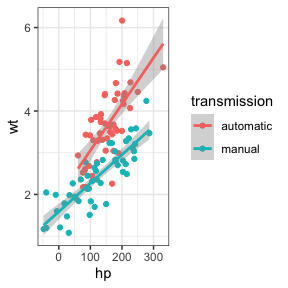Simulated iris dataset

## Empirical

Set empirical = TRUE to return a data frame with exactly the same means, SDs, and correlations as the original dataset.

exact_mtcars <- sim_df(mtcars, 50, between = "am", empirical = TRUE)

## Rounding

For now, the function only creates new variables sampled from a continuous normal distribution. I hope to add in other sampling distributions in the future. So you’d need to do any rounding or truncating yourself.

sim_df(mtcars, 50, between = "am") %>%
mutate(hp = round(hp),
transmission = factor(am, labels = c("automatic", "manual"))) %>%
ggplot(aes(hp, wt, color = transmission)) +
geom_point() +
geom_smooth(method = "lm", formula = "y~x")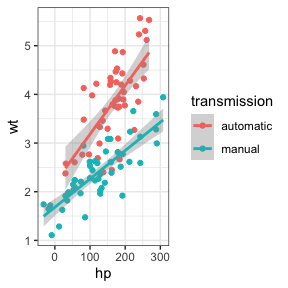Simulated iris dataset (rounded)

## Missing data

As of faux 0.0.1.8, if you want to simulate missing data, set missing = TRUE and sim_df will simulate missing data with the same joint probabilities as your data. In the dataset below, in condition B1a, 30% of W1a values are missing and 60% of W1b values are missing. This is correlated so that there is a 100% chance that W1b is missing if W1a is. There is no missing data for condition B1b.

data <- sim_design(2, 2, n = 10, plot = FALSE)
data$W1a[1:3] <- NA data$W1b[1:6] <- NA
data
#>     id  B1     W1a     W1b
#> 1  S01 B1a      NA      NA
#> 2  S02 B1a      NA      NA
#> 3  S03 B1a      NA      NA
#> 4  S04 B1a -0.8758      NA
#> 5  S05 B1a  0.2793      NA
#> 6  S06 B1a  0.4628      NA
#> 7  S07 B1a -0.1168 -0.6680
#> 8  S08 B1a  1.3445  2.3040
#> 9  S09 B1a -1.2677  0.5574
#> 10 S10 B1a -0.7126  0.0918
#> 11 S11 B1b -0.3961  0.8502
#> 12 S12 B1b -1.1536 -0.1801
#> 13 S13 B1b -0.2153  1.0887
#> 14 S14 B1b -0.4237  0.9481
#> 15 S15 B1b -0.0572  0.6367
#> 16 S16 B1b -0.1273 -1.4733
#> 17 S17 B1b  0.2121  0.6901
#> 18 S18 B1b -0.2040 -1.0106
#> 19 S19 B1b -1.1489 -0.5013
#> 20 S20 B1b  0.8759  1.4761

The simulated data will have the same pattern of missingness (sampled from the joint distribution, so it won’t be exact).

simdat <- sim_df(data, between = "B1", n = 1000,
missing = TRUE)
B1 W1a W1b n
B1a NA NA 0.31
B1a not NA NA 0.31
B1a not NA not NA 0.38
B1b not NA not NA 1.00